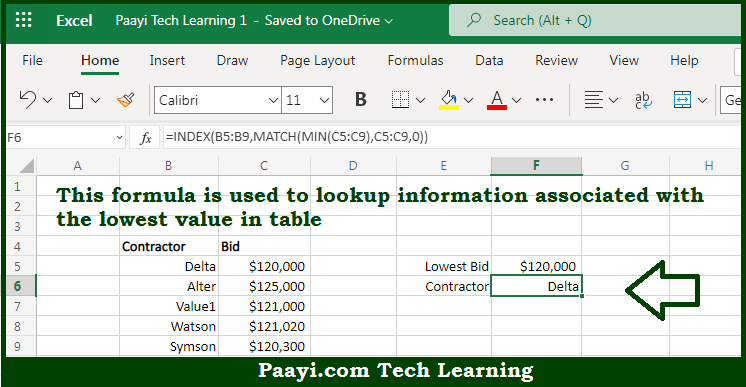# Learn How to Lookup Lowest Value in Microsoft Excel

Written by | 0 Comments | 246 Views

In this article, you will learn how to evaluate things based on the LOOKUP function in Microsoft Excel using a single/combination(s) of functions. You will also know how to Lookup Lowest Value and see the generic formula.

Learn How to Lookup Lowest Value in Microsoft Excel

The main purpose of this formula is to lookup information associated with the lowest value in the table. Here we will learn how to perform a lookup for the Lowest Value in the given data range in the workbook in Microsoft Excel. That implies, with the help of a formula based on the MIN, INDEX, and MATCH functions you can able to lookup information associated with the lowest value in the table. So, with the help of this formula, you can able to perform a lookup for the Lowest Value in the given data range in the workbook in Microsoft Excel.

General Formula to Lookup Lowest Value

=INDEX(range,MATCH(MIN(vals),vals,0))

The Explanation to Lookup Lowest ValueSo we know that with the help of the given formula above you can able to lookup information associated with the lowest value in a table. Here we will learn how to perform the lookup for the Lowest Value in the given data range in the workbook in Microsoft Excel. As we know the Match then returns the location of this value in the range, 4, which goes into INDEX as the row number along with data range in the B column as the array. So now you have learned how to perform the lookup for the Lowest Value in the given data range in the workbook in Microsoft Excel.